Home MonkeyNotes Printable Notes Digital Library Study Guides Study Smart Parents Tips College Planning Test Prep Fun Zone Help / FAQ How to Cite New Title Request

4.2 Derivability in an Interval

A function f defined on [a, b] is derivable at the end point a i.e. f  (a) exists if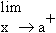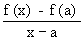exists

Similarly it is defined at the end point b, if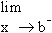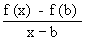if  exists.

If a function is derivable at all points of an interval except the end points a and b, it is said to be derivable in an open interval (a, b).

A function is derivable in the closed interval [a, b] if it is derivable in the open interval (a, b) and also at the end points a and b.Your browser does not support the IFRAME tag.

Example 2 Show that the function f (x) = x2 is derivable on [0, 1]

Let x0 be any point of (0, 1), then
f  (x 0 ) =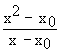=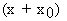= 2x0At the end points, we have

f  (0)    =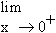=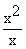=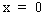and

f  (1)    =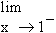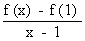=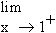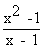=Thus the function is derivable in [0, 1].

Index

4. 1 Derivability At A Point
4. 2 Derivability In An Interval
4. 3 Derivability And Continuity Of A Function At A Point
4. 4 Some Counter Examples
4. 5 Interpretation Of Derivatives
4. 6 Theorems On Derivatives (differentiation Rules)
4. 7 Derivatives Of Standard Functions
4. 8 Derivative Of A Composite Function
4. 9 Differentiation Of Implicit Functions
4.10 Derivative Of An Inverse Function
4.11 Derivatives Of Inverse Trigonometric Functions
4.12 Derivatives Of Exponential & Logarithmic Functions
4.13 Logarithmic Differentiation
4.14 Derivatives Of Functions In Parametric Form
4.15 Higher order Derivatives

Chapter 5